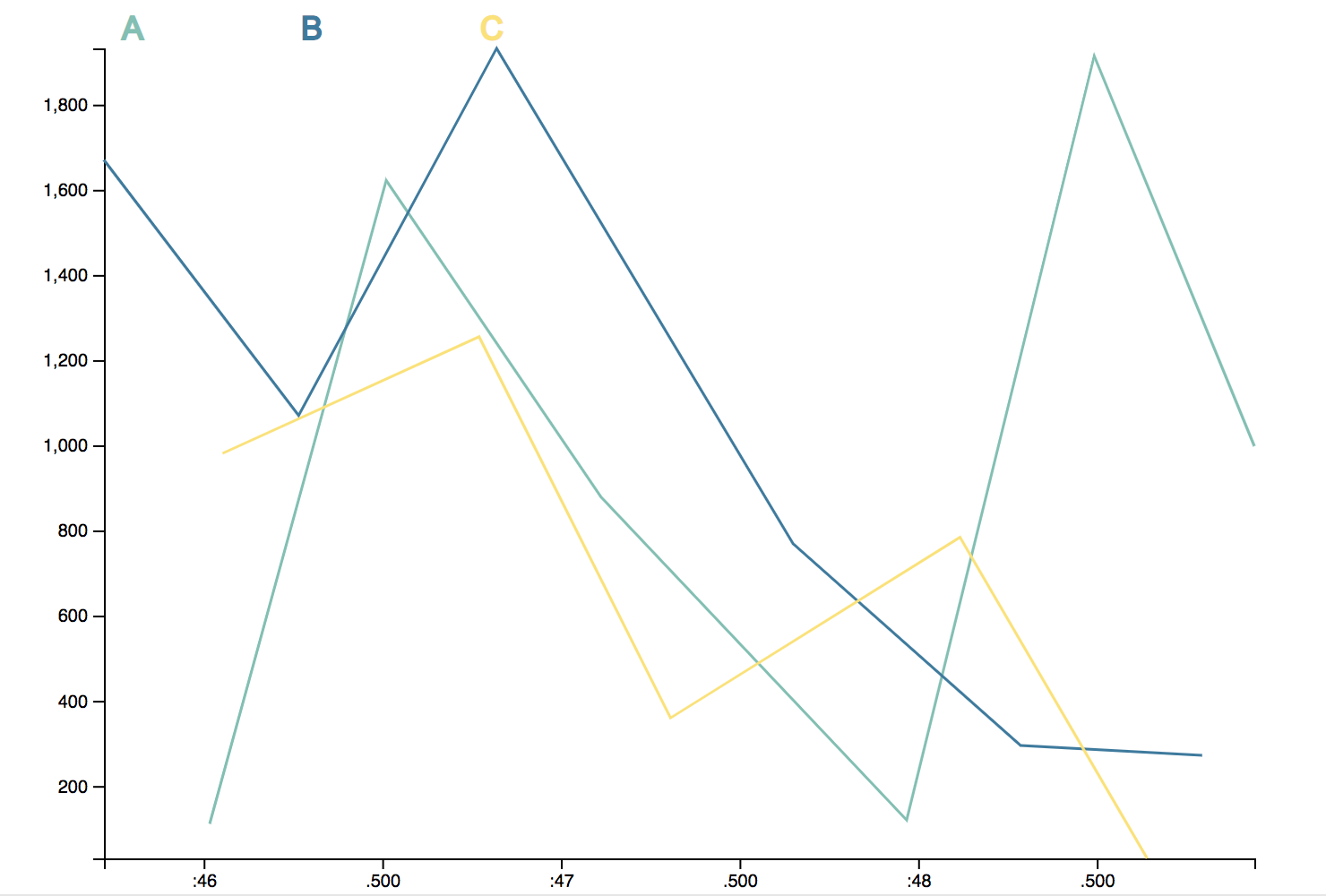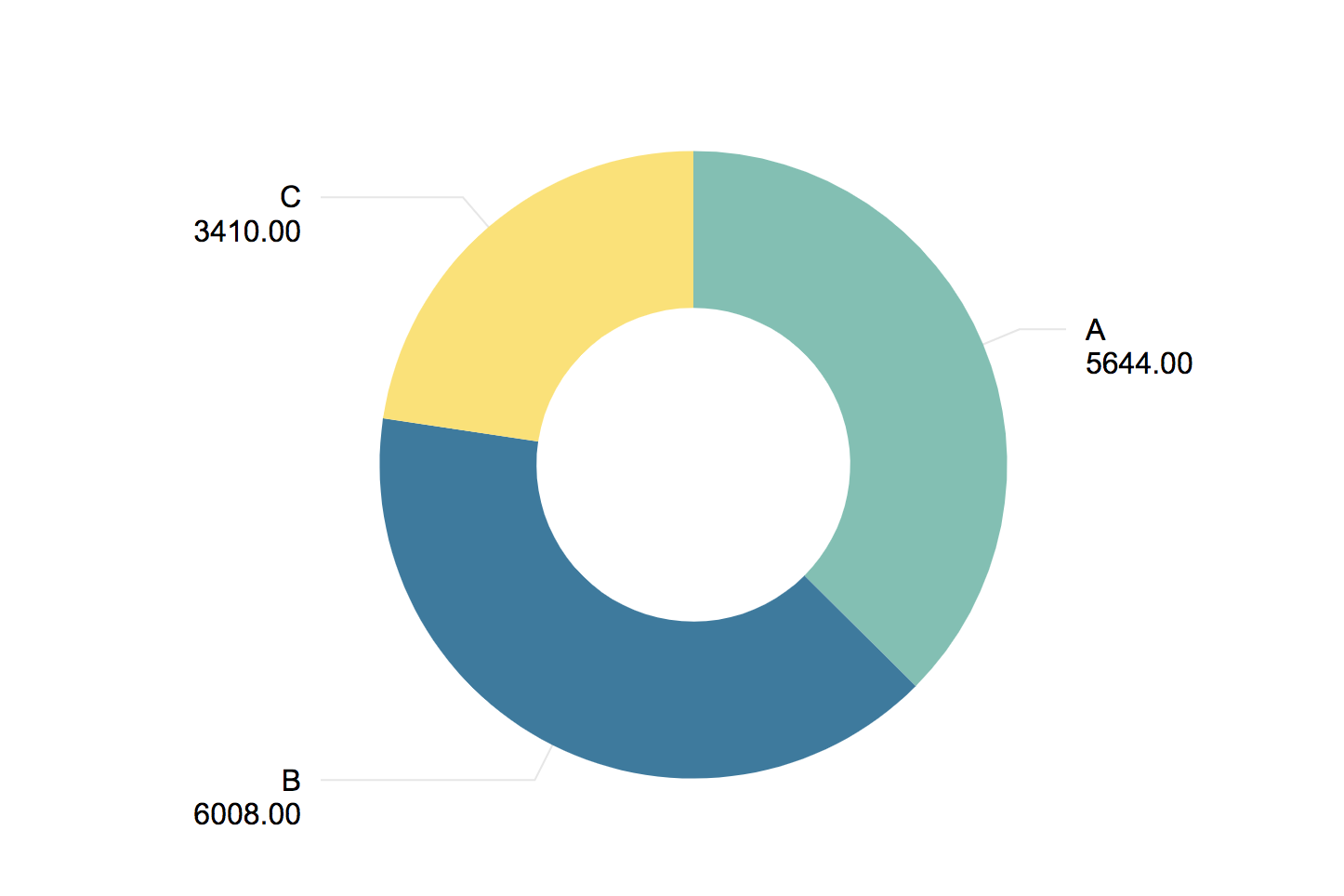# Sample InfoTypes

The following InfoTypes are free sample charts/graphs for you to test your data on.

## Line ChartDiscription: A generic line chart with x-axis data points as time and y-axis as given numerical value.

Data schema:

``````{
"id":String,
"value":Number,
"ts": UNIX timestamp
}
``````

Editable properties:

• Value to categorize data
• Value to name legened
• The amount of data to be viewed

## Bar ChartDiscription: A generic Bar chart that presents categorical data with with heights or lengths proportional to the given values.

Data schema:

``````{
"id":String,
"value":Number,
"ts": UNIX timestamp
}
``````

Editable properties:

• Label to categorize data
• Value to be represented from Labeled data

## Pie ChartDiscription: A generic pie chart dividing slices of given values into numerical proportions.

Data schema:

``````{
"id":String,
"value":Number,
"ts": UNIX timestamp
}
``````

Editable properties:

• Label to categorize data
• Value to be represented from Labeled data